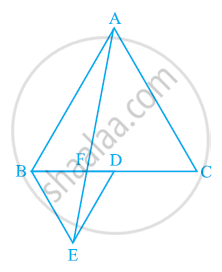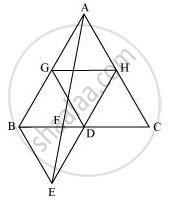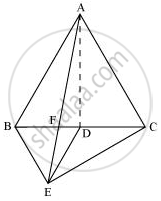# In the following figure, ABC and BDE are two equilateral triangles such that D is the mid-point of BC. If AE intersects BC at F, show that - Mathematics

In the following figure, ABC and BDE are two equilateral triangles such that D is the mid-point of BC. If AE intersects BC at F, show that(i) ar (BDE) = 1/4 ar (ABC)

(ii) ar (BDE) = 1/2 ar (BAE)

(iii) ar (ABC) = 2 ar (BEC)

(iv) ar (BFE) = ar (AFD)

(v) ar (BFE) = 2 ar (FED)

(vi) ar (FED) = 1/8 ar (AFC)

[Hint : Join EC and AD. Show that BE || AC and DE || AB, etc.]

#### Solution

(i) Let G and H be the mid-points of side AB and AC respectively.

Line segment GH is joining the mid-points. Therefore, it will be parallel to third side BC and also its length will be half of the length of BC (mid-point theorem).⇒ GH = 1/2BC and GH || BD

⇒ GH = BD = DC and GH || BD (D is the mid-point of BC)

GH ||BD and GH = BD

Two line segments joining two parallel line segments of equal length will also be equal and parallel to each other.

Therefore, BG = DH and BG || DH

Hence, quadrilateral GHDB is a parallelogram.

We know that in a parallelogram, the diagonal bisects it into two triangles of equal area.

Hence, Area (ΔBDG) = Area (ΔHGD)

Similarly, it can be proved that quadrilaterals DCHG, GDHA, and BEDG are parallelograms and their respective diagonals are dividing them into two triangles of equal area.

ar (ΔGDH) = ar (ΔCHD) (For parallelogram DCHG)

ar (ΔGDH) = ar (ΔHAG) (For parallelogram GDHA)

ar (ΔBDE) = ar (ΔDBG) (For parallelogram BEDG)

ar (ΔABC) = ar(ΔBDG) + ar(ΔGDH) + ar(ΔDCH) + ar(ΔAGH)

ar (ΔABC) = 4 × ar(ΔBDE)

Hence, ar(BDE) = 1/4 ar(ABC)

(ii) Area (ΔBDE) = Area (ΔAED) (Common base DE and DE||AB)

Area (ΔBDE) − Area (ΔFED) = Area (ΔAED) − Area (ΔFED)

Area (ΔBEF) = Area (ΔAFD) (1)

Area (ΔABD) = Area (ΔABF) + Area (ΔAFD)

Area (ΔABD) = Area (ΔABF) + Area (ΔBEF) [From equation (1)]

Area (ΔABD) = Area (ΔABE) (2)

AD is the median in ΔABC.

ar(ΔABD) = 1/2 ar(ΔABC)

= 1/4ar(ΔBDE)"                         (as proved earlier)"

ar(ΔABD) = 2ar(ΔBDE)"              ........(3)"

From (2) and (3), we obtain

2 ar (ΔBDE) = ar (ΔABE)

Or, ar(ΔBDE) = 1/2ar(ΔABE)

(iii)ar (ΔABE) = ar (ΔBEC) (Common base BE and BE||AC)

ar (ΔABF) + ar (ΔBEF) = ar (ΔBEC)

Using equation (1), we obtain

ar (ΔABF) + ar (ΔAFD) = ar (ΔBEC)

ar (ΔABD) = ar (ΔBEC)

1/2 ar(ΔABC) = ar(ΔBEC)

ar (ΔABC) = 2 ar (ΔBEC)

(iv) It is seen that ΔBDE and ar ΔAED lie on the same base (DE) and between the parallels DE and AB.

∴ ar (ΔBDE) = ar (ΔAED)

⇒ ar (ΔBDE) − ar (ΔFED) = ar (ΔAED) − ar (ΔFED)

∴ ar (ΔBFE) = ar (ΔAFD)

(v) Let h be the height of vertex E, corresponding to the side BD in ΔBDE.

Let H be the height of vertex A, corresponding to the side BC in ΔABC.

In (i), it was shown that ar(BDE) = 1/4ar(ABC).

therefore1/2xxBDxxh=1/4(1/2xxBCxxH)

rArrBDxxh=1/4(2BDxxH)

rArrh=1/2H

In (iv), it was shown that ar (ΔBFE) = ar (ΔAFD).

∴ ar (ΔBFE) = ar (ΔAFD)

=1/2xxFDxxH=1/2xxFDxx2h=2(1/2xxFDxxh)

= 2 ar (ΔFED)

"Hence, "ar(BFE) = 2ar(FED)

(vi) Area (AFC) = area (AFD) + area (ADC)

=ar(BFE)+1/2ar(ABC)"             "["In (iv), "ar(BFE) = ar(AFD);" AD is median of ΔABC"]

=ar(BFE)+1/2xx4ar(BDE)"        "["In (i), "ar(BDE)=1/4ar(ABC)]"                    Now, by (v),"

= ar(BFE) + 2ar(BDE)            ............(5)

ar(BFE) = 2ar(FED)        ...........(6)

ar(BDE) = ar(BFE) + ar(FED) = 2ar(FED) + ar(FED) = 3ar(FED)                 .........(7)

Therefore, from equations (5), (6), and (7), we get:

ar(AFC) = 2ar(FED) + 2 x 3ar(FED) = 8ar(FED)

∴ ar(AFC) = 8ar(FED)

Hence, ar(FED) = 1/8ar(AFC)

Concept: Corollary: Triangles on the same base and between the same parallels are equal in area.
Is there an error in this question or solution?
Chapter 9: Areas of Parallelograms and Triangles - Exercise 9.4 [Page 165]

#### APPEARS IN

NCERT Class 9 Maths
Chapter 9 Areas of Parallelograms and Triangles
Exercise 9.4 | Q 5 | Page 165

Share